# 2 1 Solving OneStep Equations Equivalent equations equations

• Slides: 162 -1 Solving One-Step EquationsEquivalent equations: equations that have the same solution(s) Addition Property of Equality: adding the same number to each side of the equation produces an equivalent equation Subtraction Property of Equality: Subtracting the same number from each side of an equation produces an equivalent equation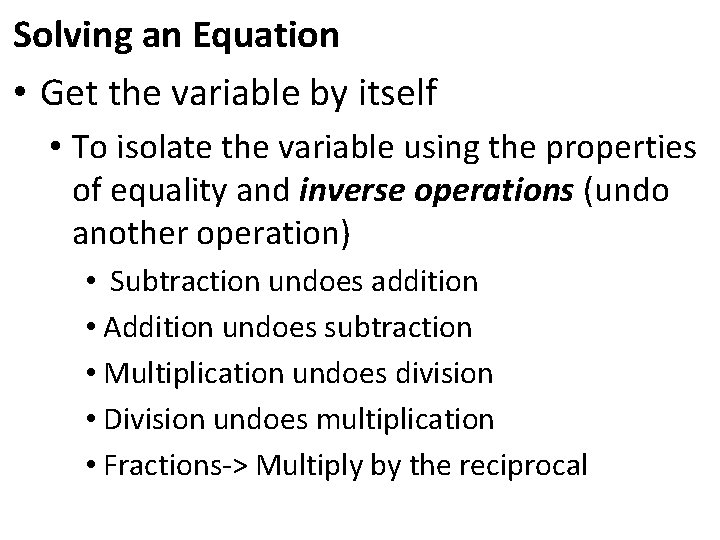Solving an Equation • Get the variable by itself • To isolate the variable using the properties of equality and inverse operations (undo another operation) • Subtraction undoes addition • Addition undoes subtraction • Multiplication undoes division • Division undoes multiplication • Fractions-> Multiply by the reciprocal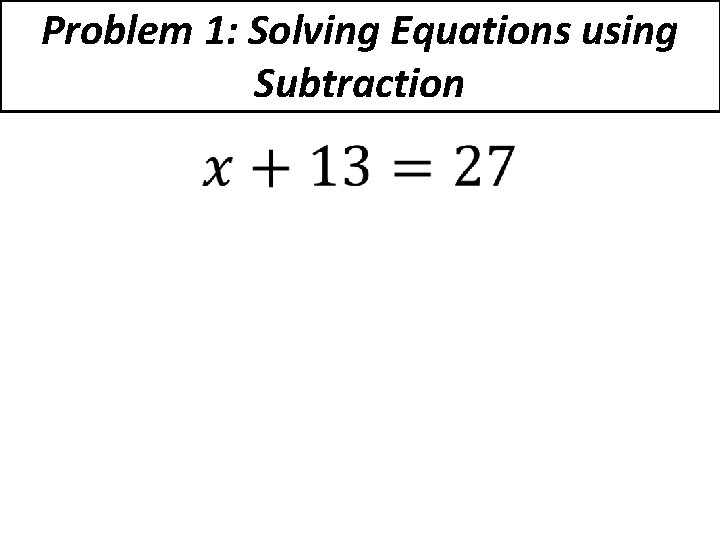Problem 1: Solving Equations using Subtraction •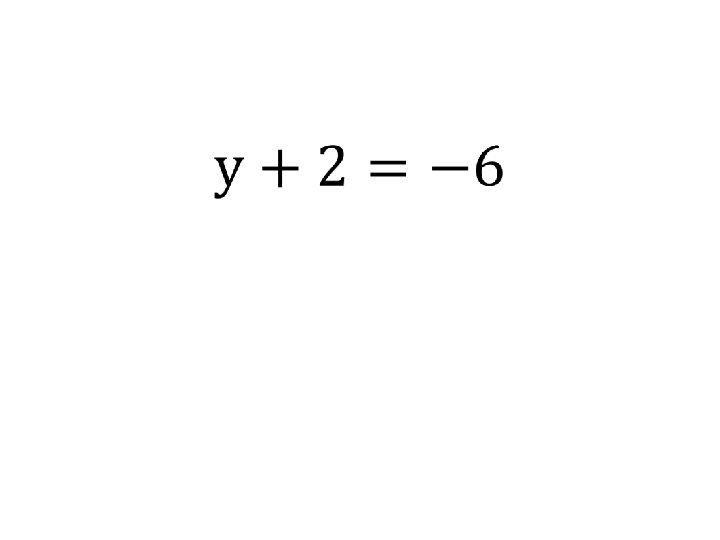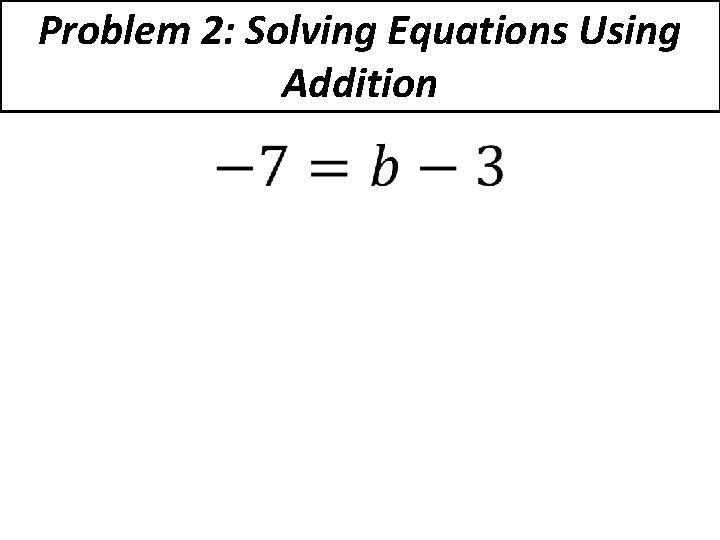Problem 2: Solving Equations Using Addition •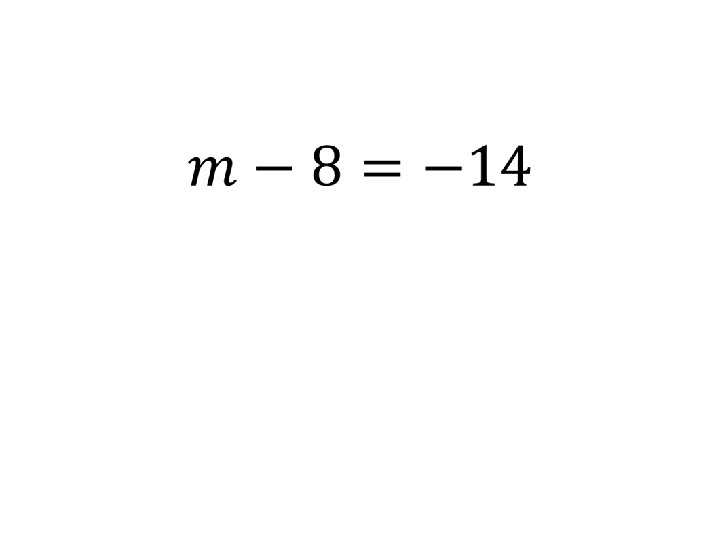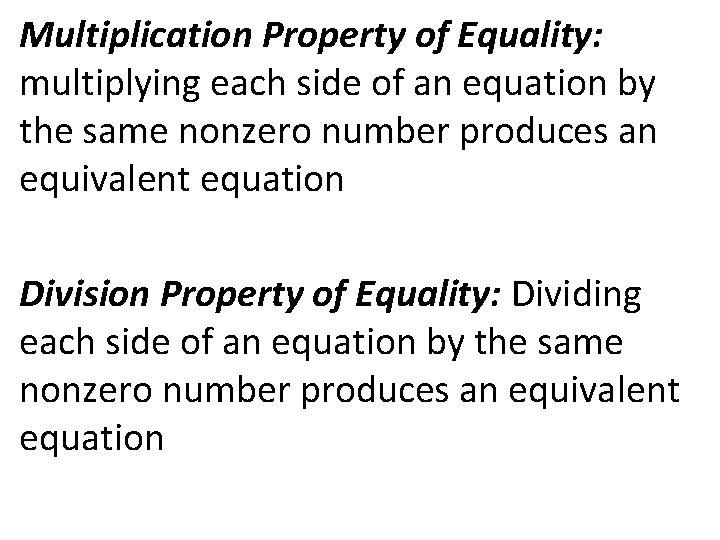Multiplication Property of Equality: multiplying each side of an equation by the same nonzero number produces an equivalent equation Division Property of Equality: Dividing each side of an equation by the same nonzero number produces an equivalent equation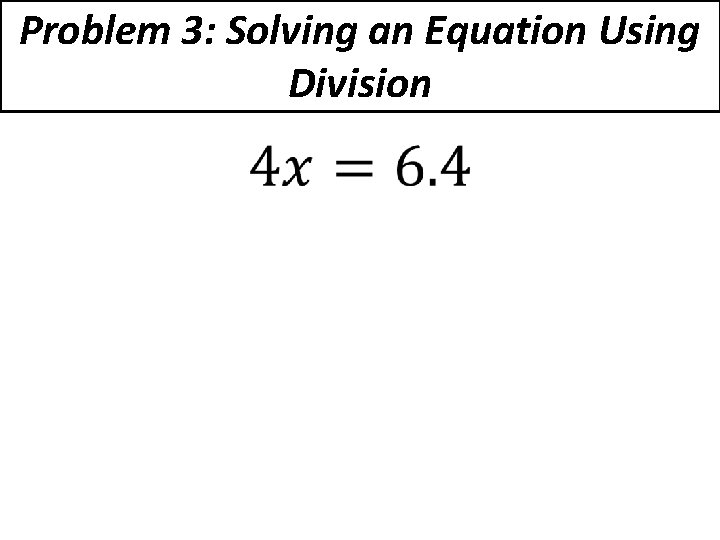Problem 3: Solving an Equation Using Division •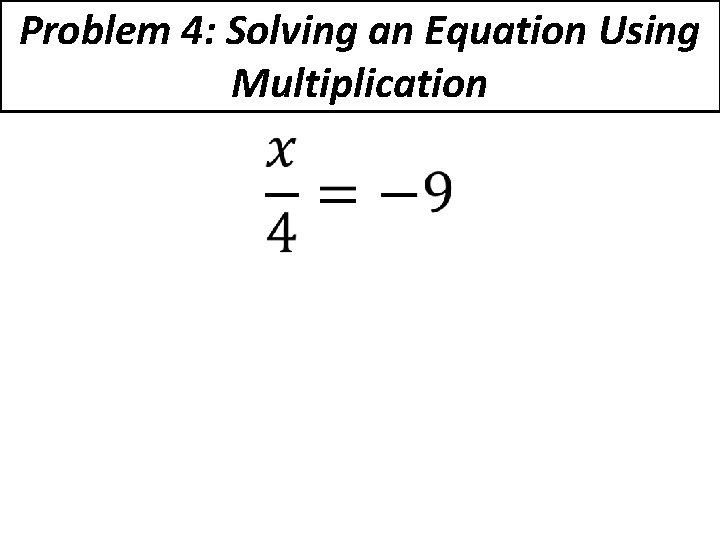Problem 4: Solving an Equation Using Multiplication •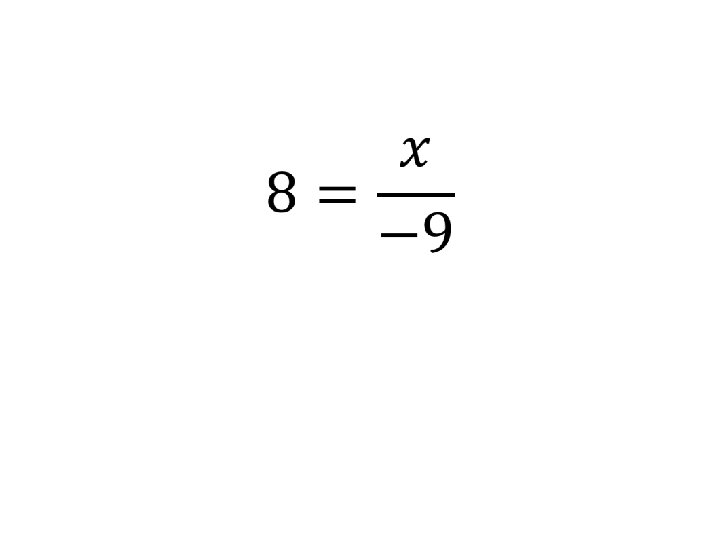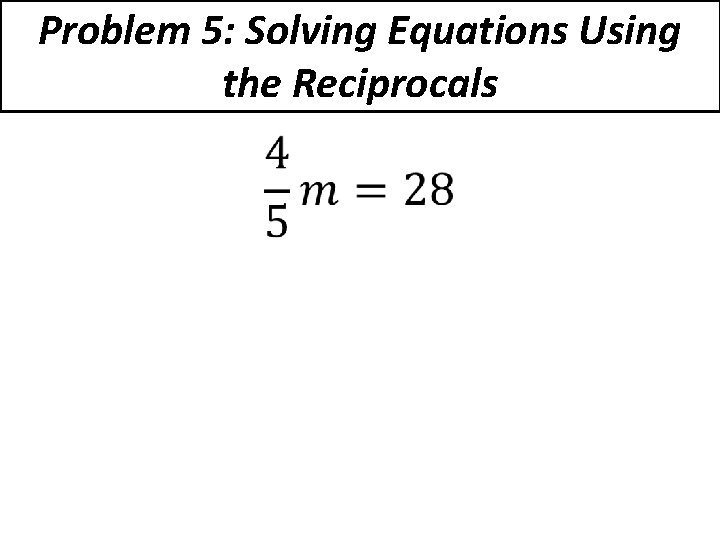Problem 5: Solving Equations Using the Reciprocals •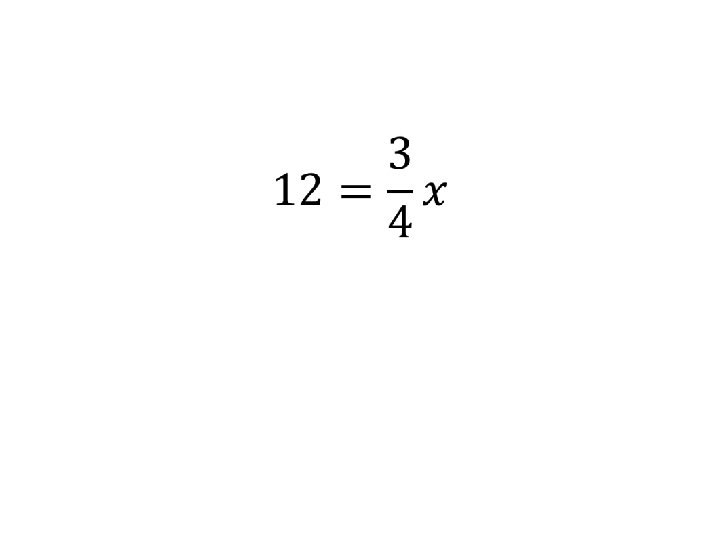Problem 6: Word Problems An online DVD rental company offers gift certificates that you can use to purchase rental plans. You have a gift certificate for \$30. The plan you select costs \$5 per month. Write and solve an equation to represent how many months can you purchase with the gift card.Toucans and macaws are both tropical birds. The length of an average toucan is about two thirds of the length of an average macaw. Toucans are about 24 inches long. Write and solve an equation to answer what is the length of the average macaw?